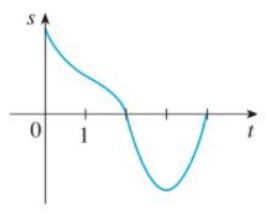Chapter 3.7, Problem 6E### Single Variable Calculus: Early Tr...

8th Edition
James Stewart
ISBN: 9781305270343

#### Solutions

Chapter
Section### Single Variable Calculus: Early Tr...

8th Edition
James Stewart
ISBN: 9781305270343
Textbook Problem

# Graphs of the position functions of two particles are shown, where 1 is measured in seconds. When is each particle speeding up? When is it slowing down? Explain.(a)(b)(a)

To determine

To find: When the particle is speeding up and slowing down.

Explanation

The given figure represents the position function of the particle.

It is identified from the graph that the position of the function s increases on the intervals (0, 1) and (3, 4) and s decreases on the interval (1, 3).

Velocity is positive when the position of the particle increases and the velocity is negative when the position of the particle decreases.

Moreover the acceleration is positive when the graph of the position of the particle is concave upward and it is negative when the curve is convex downward.

Thus, observe the following information.

The velocity is positive on the intervals (0, 1) and (3, 4) and the acceleration is positive on the interval (2, 4)...

(b)

To determine

To find: When the particle is speeding up and slowing down.

### Still sussing out bartleby?

Check out a sample textbook solution.

See a sample solution

#### The Solution to Your Study Problems

Bartleby provides explanations to thousands of textbook problems written by our experts, many with advanced degrees!

Get Started